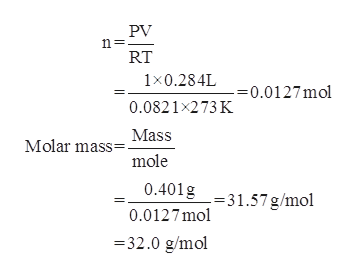# At STP, 0.284 L of a gas weighs 0.401 g. Calculate the molar mass of the gas.

Question
6 views

At STP, 0.284 L of a gas weighs 0.401 g. Calculate the molar mass of the gas.

check_circle

Step 1

Ideal gas equation is,

Step 2

Given :

Volume of gas = 0.284

Mass of gas = 0.401 g

Temperature = 273 K

Pressure = 1 atm

R = 0.082.

Su...help_outlineImage TranscriptionclosePV n= RT 1x0.284L =0.0127mol 0.0821x273 K Mass Molar mass= mole 0.401g -31.57 g/mol 0.0127mol -32.0 g/mol fullscreen

### Want to see the full answer?

See Solution

#### Want to see this answer and more?

Solutions are written by subject experts who are available 24/7. Questions are typically answered within 1 hour.*

See Solution
*Response times may vary by subject and question.
Tagged in

### Gas laws